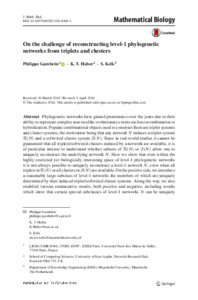# On the challenge of reconstructing level-1 phylogenetic networks from triplets and clusters

Gambette, Philippe, Huber, K. T. and Kelk, S. (2017) On the challenge of reconstructing level-1 phylogenetic networks from triplets and clusters. Journal of Mathematical Biology, 74 (7). 1729–1751. ISSN 0303-6812Preview PDF (Published manuscript) - Published Version Available under License Creative Commons Attribution. Download (811kB) | Preview

## Abstract

Phylogenetic networks have gained prominence over the years due to their ability to represent complex non-treelike evolutionary events such as recombination or hybridization. Popular combinatorial objects used to construct them are triplet systems and cluster systems, the motivation being that any network $N$ induces a triplet system $\mathcal R(N)$ and a softwired cluster system $\mathcal S(N)$. Since in real-world studies it cannot be guaranteed that all triplets/softwired clusters induced by a network are available, it is of particular interest to understand whether subsets of $\mathcal R(N)$ or $\mathcal S(N)$ allow one to uniquely reconstruct the underlying network $N$. Here we show that even within the highly restricted yet biologically interesting space of level-1 phylogenetic networks it is not always possible to uniquely reconstruct a level-1 network $N$\kelk{,} even when all triplets in $\mathcal R(N)$ or all clusters in $\mathcal S(N)$ are available. On the positive side, we introduce a reasonably large subclass of level-1 networks the members of which are uniquely determined by their induced triplet/softwired cluster systems. Along the way, we also establish various enumerative results, both positive and negative, including results which show that certain special subclasses of level-1 networks $N$ can be uniquely reconstructed from proper subsets of $\mathcal R(N)$ and $\mathcal S(N)$. We anticipate these results to be of use in the design of algorithms for phylogenetic network inference.View Item